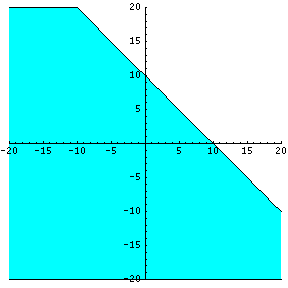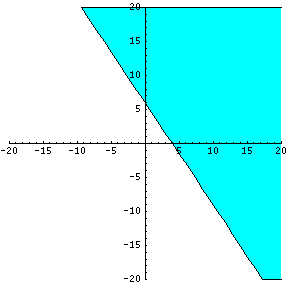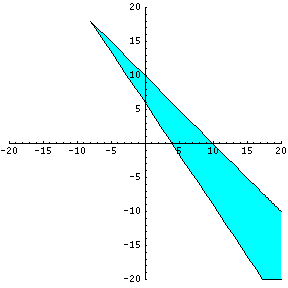# Solving Systems of Inequalities

#### We first need to review the symbols for inequalities:

• The symbol < means less than.
• The symbol > means greater than.
• The symbol $$\leq$$ means less than or equal to. Usually this is written as <= on computers because it is easier to type.
• The symbol $$\geq$$ means greater than or equal to. Sometimes this is written as >= on computers because it is easier to type.

There are endless solutions for inequalities. In light of this fact, it may be easiest to find a solution set for inequalities by solving the system graphically.

### How To Solve Systems of Inequalities Graphically

1) Write the inequality in slope-intercept form or in the form $$y = mx + b$$.

For example, if asked to solve $$x + y \leq 10$$, we first re-write as $$y \leq -x + 10$$.

2) Temporarily exchange the given inequality symbol (in this case $$\leq$$) for just equal symbol. In doing so, you can treat the inequality like an equation. BUT DO NOT forget to replace the equal symbol with the original inequality symbol at the END of the problem!

So, $$y \leq -x + 10$$ becomes $$y = -x + 10$$ for the moment.

3) Graph the line found in step 2. This will form the "boundary" of the inequality -- on one side of the line the condition will be true, on the other side it will not. Review how to graph a line here.

4) Revisit the inequality we found before as $$y \leq -x + 10$$. Notice that it is true when y is less than or equal to. In step 3 we plotted the line (the equal-to case), so now we need to account for the less-than case. Since y is less than a particular value on the low-side of the axis, we will shade the region below the line to indicate that the inequality is true for all points below the line:5) Verify. Plug in a point not on the line, like (0,0). Verify that the inequality holds. In this case, that means $$0 \leq -0+10$$, which is clearly true. We have shaded the correct side of the line.

### Example:

Find all values of x and y that satisfy: $$y \geq \frac{-3}{2}x + 6$$.

Notice that this inequality is already in the slope-intercept form. I will replace the given inequality symbol for the equal symbol to plot the line.

$$y \geq \frac{-3}{2}x + 6$$ becomes $$y = \frac{-3}{2}x + 6$$. Now plot that line as shown:Since this is a case where the inequality is true for y values greater than or equal to something, we have shaded the area above the line. All points on or ABOVE this graph line will satisfy our inequality. Again, select any point above the graph line to make sure that it will satisfy or reveal a TRUE statement in terms of the original inequality. For example, (5,3). Plug that in and we have $$3 \geq \frac{-3}{2}*5+6$$. Simplify it to $$3 \geq -1.5$$ and we see that the inequality is true at the point (5,3). Since that point was above our line, it should be shaded, which verifies our solution.

### Multiple inequalities - a system of inequalities

A system of inequalities has more than one inequality statement that must be satisfied. Graphically, it means we need to do what we just did -- plot the line represented by each inequality -- and then find the region of the graph that is true for BOTH inequalities. For the two examples above, we can combine both graphs and plot the area shared by the two inequalities.What is the solution set? The solution set for BOTH inequalities will be ANY POINT where BOTH regions are shaded together or where BOTH shaded regions meet.

Originally by Mr. Feliz, © 2005